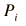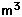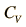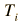# Constant Volume ProcessFor a mass of gas consisting of n= moles at a pressure= kPa = x10^ Pa,
(One atmosphere of pressure is about 101.3 kPa.)
 volume=and specific heat= J/mol K,

the increase in pressure to= kPa = x10^ Pa,

requires input heat= J = x10^ J

All of this heat contributes to the increase in the internal energy of the gas since no work is done. The initial and final temperatures can be calculated from the pressure and volumes using the ideal gas law:= K= K
Index

Heat engine concepts

 HyperPhysics***** Thermodynamics R Nave
Go Back